##### Actions

(diff) ← Older revision | Latest revision (diff) | Newer revision → (diff)

Consider a complex power series(1)

and let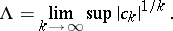If, then the series (1) is convergent only at the point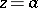; if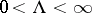, then the series (1) is absolutely convergent in the discwhere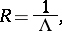(2)

and divergent outside the disc, where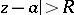; if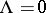, the series (1) is absolutely convergent for all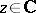. The content of the Cauchy–Hadamard theorem is thus expressed by the Cauchy–Hadamard formula (2), which should be understood in this context in a broad sense, including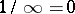and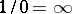. In other words, the Cauchy–Hadamard theorem states that the interior of the set of points at which the series (1) is (absolutely) convergent is the disc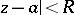of radius (2). In the case of a real power series (1), formula (2) defines the "radius" of the interval of convergence: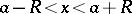. Essentially, the Cauchy–Hadamard theorem was stated by A.L. Cauchy in his lectures  in 1821; it was J. Hadamard  who made the formulation and the proof fully explicit.

For power series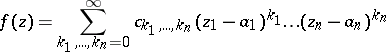(3)

incomplex variables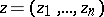,, one has the following generalization of the Cauchy–Hadamard formula: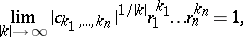(4)which is valid for the associated radii of convergenceof the series (3) (see Disc of convergence). Writing (4) in the form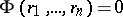, one obtains an equation defining the boundary of a certain logarithmically convex Reinhardt domain with centre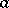, which is the interior of the set of points at which the series (3) is absolutely convergent ().

How to Cite This Entry: